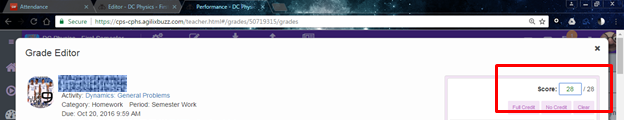Buzz: Product Feedback

Scoring Practice Question assignments

Follow

Suggestion for improvement:

When I go in to grade a Practice Questions assignment that a student has only partially completed, the score at the top is what the "weighted" score is.  This makes it difficult to grade when I don't know what the "actual" score should be.

So for instance, this assignment is set to have a weighted score of 20.This student hasn't completed all the questions, so her score is out of the weight score of 20.In order to grade the assignment, I have to go to a student who has completed the assignment and see what the total actual score is.  This student below completed all the questions showing that the assignment was actually out of 28 points.So now I go back into the original student's assignment, count up the points that she had correct, divide them by 28 and then times them by 20 to put in her score.

This would be so much easier if her assignment would just have said 0/28 to begin with.  In the event, that different students get different questions with different point values, when the student clicks on "Prepare Questions," Buzz should know what the actual total score is for each student anyway and be able to reflect that at the top of the assignment, not the weighted score.